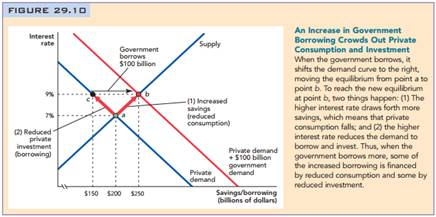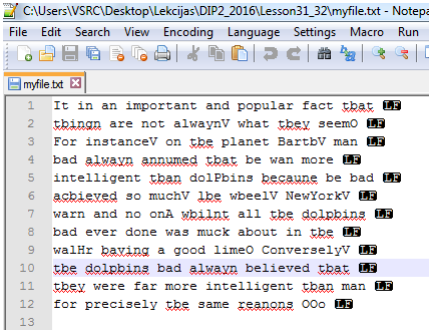### Describe how to calculate standard deviation and entropy

Assignment Help Other Engineering
##### Reference no: EM131059329

Digital Image Processing Home Work

"Representation and Description"

1. What is a descriptor?

2. Describe how to calculate following regional descriptors and give an example what objects could be well described by these descriptors (like average R,G,B values could be used to separate tomatoes from cucumbers in true color images):

2.1. standard deviation

2.2. entropy

2.3. uniformity

2.4. flatness of histogram

2.5. region area

2.6. eccentricity

Test image: example2.png

Prepare Matlab GUI for decriptor testing.1. Button Load I from workspace: loads RGB image called I from Matlab workspace and shows it in axes 1.

2. Button Load BW from workspace: loads segmented image BW by using thresholding and shows it in axes 2. Improve the result of thresholding by removing small meaningless components using morphological processing.

3. Once both images are loaded, then find connected components and show them in axes3.

4. User can click on connected components in axes3. Selected connected component will be shown in axes4 and following descriptors of the region will be calculated and shown in Static Text object:

a) mean R value of Connected Component (CC);

b) mean G value of CC

c) mean B value of CC

d) standard deviation of CC

e) eccentricity of CC

f) entropy of CC

g) area of CC.

"Object Recognition"

T1: OPTICAL CHARACTER RECOGNITION

Perform character recognition in the image and write text to the file.

Test images: TMR.jpg, hitchickersGuideToTheGalaxy.jpg

Procedure:

1. Write a function findRows() which can separate line of the text in the image and save all lines as images in a cell array.

2. Prepare sample data. Import image TMR.jpg, convert it to binary image, invert it and smooth the boundaries by using imclose.

2.1. Extract lines and separate each symbol by using bwlabel.

2.2. Extract each symbol as im using regionprops. Calculate signature sy for this symbol using prepareSignature(). Write in cell array templateset: im, sy and name of the symbol (idea char('A'+(1:26)-1)' could be useful).3. Process text in hitchhickersGuideToTheGalaxy.jpg: convert it to binary image, invert it and smoothe the boudaries by using imclose.

3.1. Extract lines of text.

3.2. For each line

3.2.1. Find all symbols using bwlabel.

3.2.2. Calculate signature for each symbol and compare this signature with all signatures in templateset (you can use Euclidean distance or mean absolute difference). Assign symbol to the character of template set with the most similar signature.

3.2.3. Write each line in .txt file

4. Write a function findWords for finding words in the line of the text (in the image).

5. Add word separation to the code above and write white spaces in the text file as well.#### One drawback for application that polypyrrole very fragile

Calculate the current generated in a 10-mm-long, 10.0 × 10-6-m-diameter polypyrrole filament that is subjected to a electric field gradient of 12 V. Assume that the conducti

#### Draw the logic diagram for the hierarchical component

Three instances (copies) of a single hierarchical circuit component made up of two AND gates, an OR gate, and an inverter are to be used. Draw the logic diagram for the hier

#### Testing of a new lubricant in cold metal forming

ENGG851 - Assessment - The abstract should be no longer than 200 words. While the abstract is the first activity it should be the very last thing that you write. If you t

#### Determining the pressure in atmospheres

Hydraulic Lift II The piston of a hydraulic automobile lift is 0.30 m in diameter. What gauge pressure, in Pascal's, is required to lift a car with a mass of 1200 kg? Also e

#### Give a brief notes on system load variation

Give a brief notes on the following topics. 1. System load variation: a. System load characteristics b. load curves c. load duration curve d. load factor e. Diversity factor 2

#### Develop an influence diagram and a decision tree

Develop an influence diagram and a decision tree for this situation. What roles do the two diagrams play in helping to understand and communicate the structure of this decis

#### Design the appropriate architecture for the project

You are required to design an energy meter based on CPLD (Complex Programmable Logic Device) that will smartly disconnect the supply when sufficient amount of energy units are

#### Create an edge-triggered d flip-flop

To simulate the operation of a circuit used to create an edge-triggered D flip-flop. To test the operation of a 74LS74 D flip-flop. To test the operation of a 74LS112 J-K flip

### Write a Review